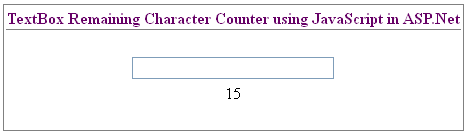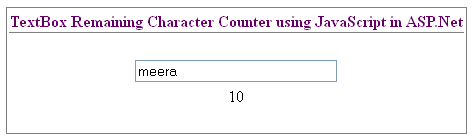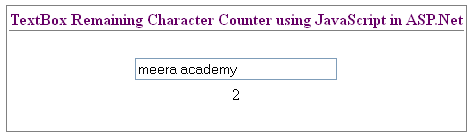# TextBox Remaining Character Counter using JavaScript in ASP.Net

In this asp.net post i will show you how to count the remaining character in textbox control using javaScript in asp.net.

First design asp.net web forms with one textbox control and a Label Control.

Here, we can see the remaining character while we write text in textbox control, we use javaScript for count the remaining character.

Here, we call the javascript function on onkeyup textbox events.

First set the textbox MaxLength=15 and call javascript function on onkeyup=”cnt()”.

below is the design of asp.net web forms:

Here is the JavaScript function for count remaining character:

The output of count remaining character in textbox using javascript :TextBox Remaining Character Counter using JavaScript in ASP.Net

– above screen shows the total length of textbox is 15. when we write text into textbox the count automatically decreases according to length of textbox text.TextBox Remaining Character Counter using JavaScript in ASP.Net

– in above forms we write “meera” the 5 character then the count shows the 10 character is remaining in textbox control.TextBox Remaining Character Counter using JavaScript in ASP.Net